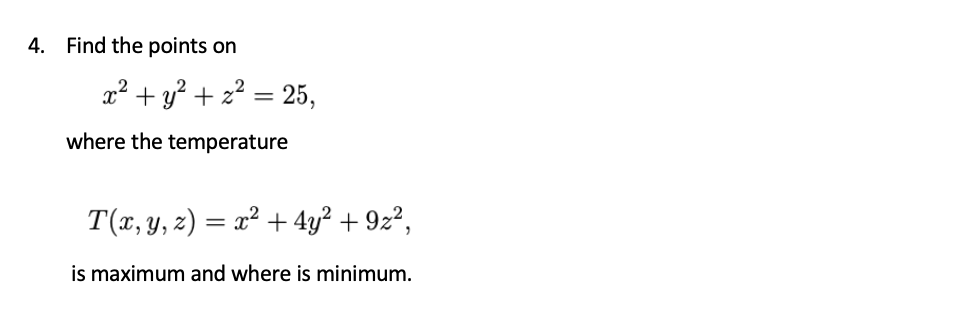# 4. Find the points on x² + y? + z? = 25, where the temperature T(x, y, z) = x² + 4y² + 9z², %3D is maximum and where is minimum.

Questionhelp_outlineImage Transcriptionclose4. Find the points on x² + y? + z? = 25, where the temperature T(x, y, z) = x² + 4y² + 9z², %3D is maximum and where is minimum. fullscreen

### Want to see this answer and more?

Experts are waiting 24/7 to provide step-by-step solutions in as fast as 30 minutes!*

*Response times vary by subject and question complexity. Median response time is 34 minutes and may be longer for new subjects.
Tagged in
MathCalculus

### Applications of Derivative